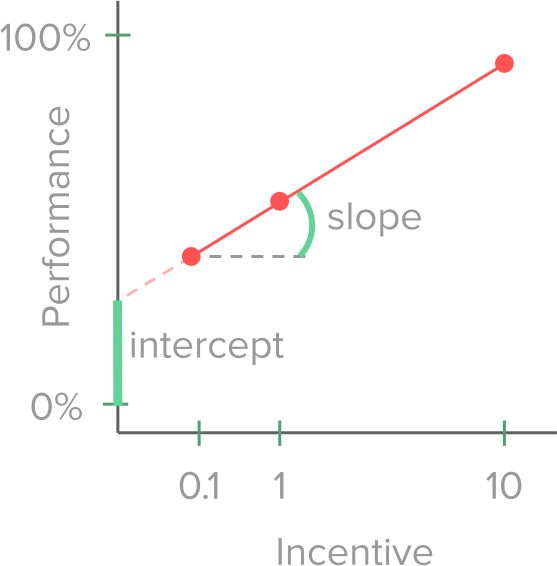top of page
• antoniuswiehler

# Orthogonalization

Why do we care about orthogonalization? This is a fix for a problem which is called (multi-)collinearity. Linear regression (a general linear model, GLM) only works correctly, when its regressors are not correlated (not correlated = orthogonal). In the best case, this has been considered during the designing of the experiment, so that all factors vary independently of each other. However, it still can be that some regressors are at least partially correlated and that creates problems for the regression. You can think of it as if the regression “does not know” which estimate to assign to which regressor because multiple regressors explain the same part of the variance.

I suggest having a look at chapter 5.3 (“When adding variables hurts”) in this book by Richard McElreath. It is extremely well written and explains the problem greatly!

So, imagine the regressors are (partially) correlated, what can we do to fix the problem? This is the call for orthogonalization. First, You need to decide on an order of regressors. Which are the regressors you think will explain most of the signal? For which regressors would you like to control your analysis? These regressors go first.

Second, you regress the regressors against each other, in that given order. First, the second regressor will be predicted by the first regressor. If the regressors are correlated, the first regressor explains a lot of variance of the second regressor. We keep the residuals (the part of the second regressor that is not explained by the first regressor) as the unique signal of the second regressor. The residuals of the second regressor are orthogonal to the first regressor (hence the name orthogonalization). Next, the third regressor will be predicted by the first regressor and the residuals of the second regressor, and only the residuals of the third regressor are kept. And so on…This leads to regressors that are not correlated, which is great. However, when regressors have been highly correlated, this usually does not leave much after cleaning (this is the reason why we want to take care of that already during the design of the experiment and make sure they are as little correlated as possible).

In the VBA toolbox, the function VBA_orth.m is doing the trick.

You input a matrix into the function and the function orthogonalizes the columns going from left to right. So basically, it predicts the 2nd column from the first one with a regression. Then it explains column 3 by the columns left to it, and so on.

The practical thing is here, that you have to decide on the order for orthogonalization. So the regressors you care about go first (left columns in the matrix) because they get assigned the part of the variance that is shared between regressors and everything else comes after.